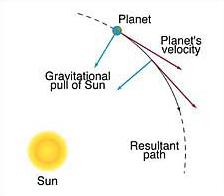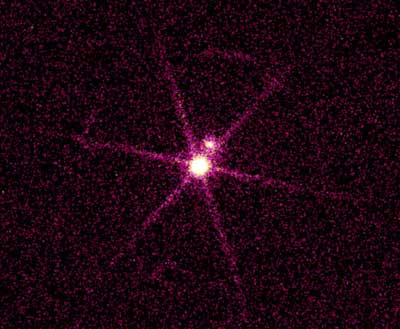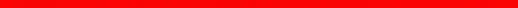Newton: Motion and GravityNewton's Laws of Motion I, Inertia: An object moves at constant velocity, unless it feels a net force. II, Force: If a net force acts, the acceleration is that is related to the force by F = ma (inversely proportional to the mass). III, Action and Reaction: The force B → A is equal and opposite to the force A → B. What is he saying? A force does not cause an object to have a velocity, but an acceleration! When two objects interact, the mutual forces are always equal, although the lighter one is more strongly affected. Also, you can't push without being pushed. So, for example: When a rocket lifts off, does it push against the ground? What keeps the Moon or a satellite moving along its orbit up there? Mass and Weight: They are not the same thing; Mass is the "amount of substance" of an object and does not change; Weight is the effect of gravity on mass (but it can appear to be zero even when there is gravity); You can be weightless, not massless.Newton's Law of Gravity The law: There is a (weak) force of gravity between any two objects, including celestial objects (there is gravity in space), and it can be calculated using the inverse-square law F = G Mm / r2. And planetary motion: Celestial objects like planets obey the same laws as earthly ones! We now understand Kepler's laws, they can be explained using the force of gravity that the Sun pulls with. New twist #1: The planets and the Sun actually orbit around their center of mass! New twist #2: There can be new types of orbits, unbound ones [hyperbolas or parabolas], in addition to ellipses. New twist #3: What relates the size and period of an orbit in Kepler's 3rd law are the masses involved. New twist #4: Planets feel each other's gravity, and that of everything else. Questions: In the real world, are the orbits of planets really ellipses? Which objects are not affected by gravity, according to Newton's theory?Other Situations and Related Concepts Acceleration of gravity: Because mass affects both the force of gravity and an object's resistance to accelerate (inertia), all objects end up falling at the same rate. Gravity on Earth: On the Earth's surface, using its mass, we get an acceleration of 9.8 m/s2.Around other objects: The laws of motion and gravity hold for any body around any gravitational center of attraction, even asteroids, binary stars and galaxies... By the way, gravity explains the fact that small moons and asteroids are not round, while larger objects are. Satellites around the Earth: Larger orbits have longer periods; e.g., geosynchronous ones [42,000 km], or 90 minutes for the Space Shuttle. Our Moon: Its orbit is elliptical too (and the period that enters into Kepler's laws is the sidereal month, rather than the lunar/synodic one!). New concept: The escape velocity from a center of attraction; depends on its mass and size [11 km/s from the Earth's surface].
 Other Consequences Tides on Earth: Every 12 hours, due to the Moon (and the Sun, so we get enhanced spring tides at full and new moon, and reduced neap tides), affecting mostly the water. [Consequences: The Earth's rotation rate is slowing down (by 1 day every 50,000 years!), and the distance to the Moon increasing.] Other tides: Our Moon's rotation (and that of other moons in the Solar System) became locked to its revolution; Comets can break into pieces when approaching planets; Extreme cases near black holes; Pairs of galaxies [tides seem to enhance star formation!].Use of the third law: One can calculate masses from sizes of orbits and periods; e.g., Jupiter and its moons, Sirius A and B; asteroid Ida and its satellite. Motion around the center of mass: In binary star systems, we often see both stars moving!page by luca bombelli <bombelli at olemiss.edu, modified 16 sep 2013